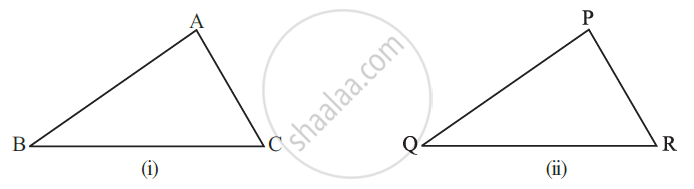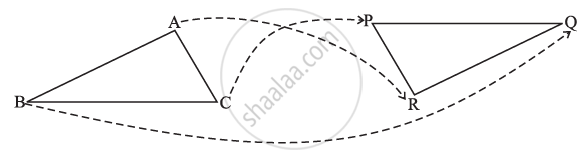# Congruence of Triangles:

Two triangles are congruent if the sides and angles of one triangle are equal to the corresponding sides and angles of the other triangle.△ ABC and △ PQR have the same size and shape. They are congruent.

△ABC ≅ △PQR

This means that, when you place △PQR on △ABC, P falls on A, Q falls on B and R falls on C, also PQ falls along bar"AB",  bar"QR"   "falls along"   bar"BC" and bar"PR"   "falls along"   bar"AC".

If, under a given correspondence, two triangles are congruent, then their corresponding parts (i.e., angles and sides) that match one another are equal.

Thus, in these two congruent triangles.

We have:

• Corresponding vertices: A and P, B and Q, C, and R.

• Corresponding sides: bar"AB" and bar"PQ", bar"BC" and bar"QR", bar"AC" and bar"PR".

• Corresponding angles: ∠A and ∠P, ∠B and ∠Q, ∠C, and ∠R.

Congruent triangles corresponding parts in short ‘CPCT’ stands for corresponding parts of congruent triangles.

## Example

∆ABC and ∆PQR are congruent under the correspondence:
ABC ↔ RQP
Write the parts of ∆ABC that correspond to
(i) bar"PQ"
(ii)∠Q
(iii) bar"RP"

For a better understanding of the correspondence, let us use a diagramThe correspondence is ABC ↔ RQP.
This means A ↔ R; B ↔ Q; and C ↔ P.
So,
(i) bar"PQ" ↔ bar"CB"
(ii) ∠Q ↔ ∠B and
(iii) bar"RP" ↔ bar"AC".

If you would like to contribute notes or other learning material, please submit them using the button below.

### Shaalaa.com

What is Congruence of Triangles? [00:10:01]
S
0%